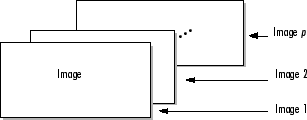## Work with Image Sequences as Multidimensional Arrays

### Create Multidimensional Array Representing Image Sequence

Multidimensional arrays are a convenient way to display and process image sequences. Create a multidimensional array by concatenating the individual images of an image sequences. Each image must be the same size and have the same number of color channels. If you are storing a sequence of indexed images, each image must use the same color map.

• If you have a sequence of 2-D grayscale, binary, or indexed images, then concatenate the images in the third dimension to create a 3-D array of size m-by-n-by-p. Each of the p images has size m-by-n.

• If you have a sequence of 2-D RGB images, then concatenate the images along the fourth dimension to create a 4-D array of size m-by-n-by-3-by-p. Each of the p images has size m-by-n-by-3.

The figure depicts 2-D images concatenated as planes of a 3-D array.Use the `cat` function to concatenate individual images. For example, this code concatenates a group of RGB images along the fourth dimension.

`A = cat(4,A1,A2,A3,A4,A5)`

Note

Some functions work with a particular type of multidimensional array, call a multiframe array. In a multiframe array, images are concatenated along the fourth dimension regardless of the number of color channels that the images have. A multiframe array of grayscale, binary, or indexed images has size m-by-n-by-1-by-p. If you need to convert a multiframe array of grayscale images to a 3-D array for use with other toolbox functions, then you can use the `squeeze` function to remove the singleton dimension.

### Display Image Sequences

There are several ways to display image sequences. To display one frame at a time, use the Image Viewer app or `imshow`. To display all the frames in an image sequence simultaneously, use the `montage` function.

To animate an image sequence or provide navigation within the sequence, use the Video Viewer app (`implay`). The Video Viewer app provides playback controls that you can use to navigate among the frames in the sequence.

### Process Image Sequences

Many toolbox functions can operate on multidimensional arrays and, consequently, can operate on image sequences. For example, if you pass a multidimensional array to the `imtransform` function, it applies the same 2-D transformation to all 2-D planes along the higher dimension.

Some toolbox functions that accept multidimensional arrays, however, do not by default interpret an m-by-n-by-p or an m-by-n-by-3-by-p array as an image sequence. To use these functions with image sequences, you must use particular syntax and be aware of other limitations. The table lists these toolbox functions and provides guidelines about how to use them to process image sequences.

Function

Image Sequence Dimensions

Guideline When Used with an Image Sequence

`bwlabeln`

m-by-n-by-p only

Must use the `bwlabeln(BW,conn)` syntax with a 2-D connectivity.

`deconvblind`

m-by-n-by-p or
m-by-n-by-3-by-p

`PSF` argument can be either 1-D or 2-D.

`deconvlucy`

m-by-n-by-p or
m-by-n-by-3-by-p

`PSF` argument can be either 1-D or 2-D.

`edgetaper`

m-by-n-by-p or
m-by-n-by-3-by-p

`PSF` argument can be either 1-D or 2-D.

`entropyfilt`

m-by-n-by-p only

`nhood` argument must be 2-D.

`imabsdiff`

m-by-n-by-p or
m-by-n-by-3-by-p

Image sequences must be the same size.

`imadd`

m-by-n-by-p or
m-by-n-by-3-by-p

Image sequences must be the same size. Cannot add scalar to image sequence.

`imbothat`

m-by-n-by-p only

`SE` argument must be 2-D.

`imclose`

m-by-n-by-p only

`SE` argument must be 2-D.

`imdilate`

m-by-n-by-p only

`SE` argument must be 2-D.

`imdivide`

m-by-n-by-p or
m-by-n-by-3-by-p

Image sequences must be the same size.

`imerode`

m-by-n-by-p only

`SE` argument must be 2-D.

`imextendedmax`

m-by-n-by-p only

Must use the `imextendedmax(I,h,conn)` syntax with a 2-D connectivity.

`imextendedmin`

m-by-n-by-p only

Must use the `imextendedmin(I,h,conn)` syntax with a 2-D connectivity.

`imfilter`

m-by-n-by-p or
m-by-n-by-3-by-p

With grayscale images, `h` can be 2-D. With truecolor images (RGB), `h` can be 2-D or 3-D.

`imhmax`

m-by-n-by-p only

Must use the `imhmax(I,h,conn)` syntax with a 2-D connectivity.

`imhmin`

m-by-n-by-p only

Must use the `imhmin(I,h,conn)` syntax with a 2-D connectivity.

`imlincomb`

m-by-n-by-p or
m-by-n-by-3-by-p

Image sequences must be the same size.

`immultiply`

m-by-n-by-p or
m-by-n-by-3-by-p

Image sequences must be the same size.

`imopen`

m-by-n-by-p only

`SE` argument must be 2-D.

`imregionalmax`

m-by-n-by-p only

Must use the `imextendedmax(I,conn)` syntax with a 2-D connectivity.

`imregionalmin`

m-by-n-by-p only

Must use the `imextendedmin(I,conn)` syntax with a 2-D connectivity.

`imsubtract`

m-by-n-by-p or
m-by-n-by-3-by-p

Image sequences must be the same size.

`imtophat`

m-by-n-by-p only

`SE` argument must be 2-D.

`imwarp`

m-by-n-by-p or
m-by-n-by-3-by-p

`TFORM` argument must be 2-D.

`padarray`

m-by-n-by-p or
m-by-n-by-3-by-p

`PADSIZE` argument must be a two-element vector.

`rangefilt`

m-by-n-by-p only

`NHOOD` argument must be 2-D.

`stdfilt`

m-by-n-by-p only

`NHOOD` argument must be 2-D.

`tformarray`

m-by-n-by-p or
m-by-n-by-3-by-p

• `T` must be 2-D to 2-D (compatible with `imtransform`).

• `R` must be 2-D.

• `TDIMS_A` and `TDIMS_B` must be 2-D, that is,`[2 1]` or ```[1 2]```.

• `TSIZE_B` must be a two-element array `[D1 D2]`, where `D1` and `D2` are the first and second transform dimensions of the output space.

• `TMAP_B` must be ```[TSIZE_B 2]```.

• `F` can be a scalar or a p-by-1 array, for m-by-n-by-p arrays. Or `F` can be a scalar, 1-by-p array; 3-by-1 array; or 3-by-p array, for m-by-n-by-3-by-p arrays.

`watershed`

m-by-n-by-p only

Must use `watershed(I,conn)` syntax with a 2-D connectivity.